Examples: Electric Field & Potential

# Examples: Electric Field & Potential - Notes | Study Electromagnetic Fields Theory (EMFT) - Electrical Engineering (EE)

 1 Crore+ students have signed up on EduRev. Have you?

More Examples  of Electric Field and Potential

Example 1: Two intersecting and oppositely charged spheres :

We consider the electric field in the region of intersection of two oppositely charged but otherwise identical spheres. The spheres are assumed to be charged uniformly.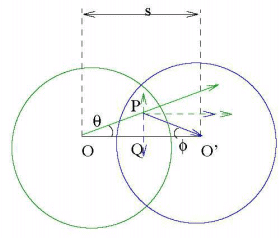We have seen that the electric field inside a uniformly charged sphere is linear in r, the distance from the centre,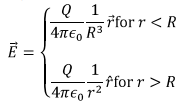Since the region of intersection is interior to both the spheres, at a point P in this region, we have,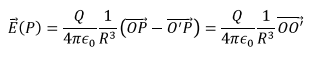It is seen that the field depends only on the distance between the two centres and is independent of position of P within this intersection.

Example 2 : Field inside a non-concentric cavity inside a uniformly charged sphere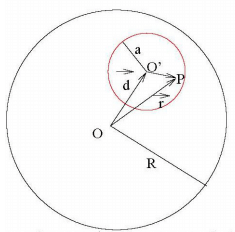Consider a sphere of radius R with its centre at O. There is an off centre cavity of radius a whosecentre is at O’.

The way to do these problems is to consider the cavity as a superposition of equal and oppositely charged spheres , the charge density of the two spheres being equal to the charge density of the charged part of the bigger sphere. This makes the problem equivalent to finding the field inside the cavity region due to a sphere of charge density with its centre at O and another sphere of charge density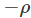with its centre at O’

Let the point P be at a position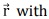respect to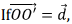then the field at P due to the bigger sphere (with density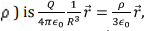while that due to the smaller sphere of charge density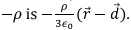Thus the net field is given by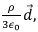which is constant inside the cavity.

Nature of Coulomb Force:

1. It is a central force, inverse square in nature.
2. Force is conservative :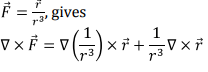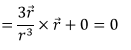3. Since the force is conservative, it can be expressed as a gradient of a scalar potential,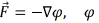is known as scalar potential.

Electrostatic Potential :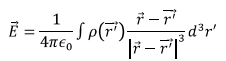Using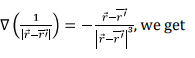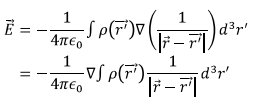The gradient operator can be taken outside as the gradient is with respect to unprimed variable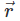,while the integration is with respect to primed variable. This gives,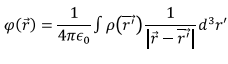Note that the divergence of the electric field can be written as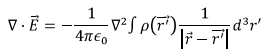TheLaplacian operator, acting on unprimed variable can be taken inside the integration,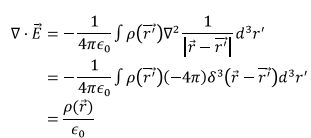Thus we have the following two relations for the electrostatic field :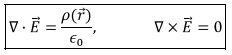Suppose you are bringing a unit charge from some reference point (where the potential is defined as zero), the work that needs to be done by you (i.e. by an external agency) to bring this charge from the reference point to a positionis negative of the work done by the force on the charge. The work done by the force is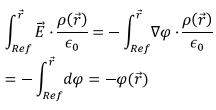For instance, for field produced by a point charge q at the origin, the potential at the point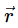is (where the reference point is taken to be at infinity)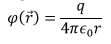The name “potential” is very similar to the phrase “potential energy” and this is often confusing because though there is a connection between the two, they are different things. The relationship is understood by considering the work that needs to be done to bring a test charge q from the reference point to the point P where it is to be placed, The work done by an external agency, as calculated above is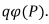This work done then becomes the potential energy of the system. Thus the potential at a point can be interpreted as the potential energy associated with a unit point charge at that point.

Poisson’s Equation

We have seen that the divergence of the electric field satisfies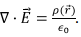Substituting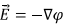in this equation, we get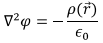This is known as the Poisson’s equation. In a region of space where there are no charges the equation satisfied by the potential is the Laplace’s equation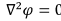Example 3: Potential due to a line charge

We have seen that the field due to a line charge can be evaluated by enclosing the line charge with a Gaussian cylinder of length l and radius r. The field can only depend on the distance from the wire and be directed away from the wire. The contribution to the flux from the top and bottom caps are zero and the flux is contributed only by the curved surface of the cylinder, giving,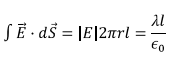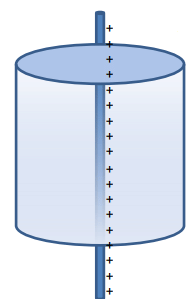which gives (plugging in the direction of the field)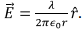Equating this to the gradient of potential, (which in this case is just the derivative with respect to r) we get,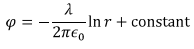Unlike in the case of a point charge, the reference point of the potential cannot be taken at infinite distances because logarithm gets undefined there. We can choose some arbitrary unit and choose the potential to be zero at r=1 and with respect to this reference point, the potential at a distance r from the line charge is given by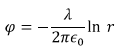Example 4 : Screened Coulomb Potential

A screened Coulomb potential, also known as Yukawa potential arises in systems such as semiconductors where the medium in which interaction takes place (a dielectric) partially screens the bare Coulomb interaction between charges. The potential is given by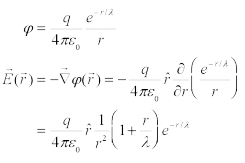The charge distribution that gives rise to this electric field can be obtained by calculating the divergence of the electric field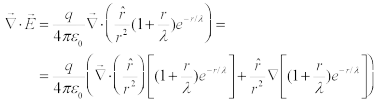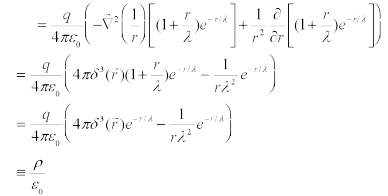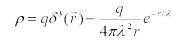which shows that there is a point charge at the origin in addition to an exponentially decaying charge density. The total charge can be obtained by integrating the charge density over the entire volume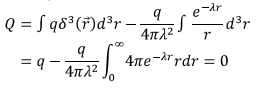What is the advantage of using the potential formulation over calculating electric field? Potential being a scalar is easier to deal with mathematically. When dealing with multiple charges, it is simpler to add up the potential using superposition principle and then take the gradient to determine the electric field. Potential is analogous to pressure in a fluid. Just as a fluid in a pipe tends to move from a region of high pressure to that of low pressure, in an electric field positive charges tend to move from a region of higher potential to that of a lower potential.

Potential and Electric Field of a dipole

A dipole is basically two equal and opposite charges separated by a distance. For an ideal dipole the separation goes to zero. Dipole moment is a vector defined as a vector of magnitude equal to the product of the magnitude of either charge and the distance between them. The direction of the dipole moment vector is defined as along the direction from the negative charge to the positive charge.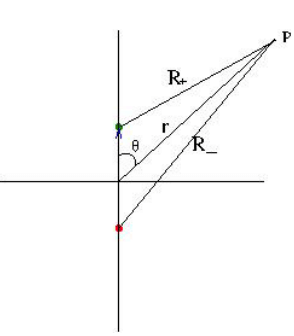In the figure the positive charge is shown by the blue circle and the negative charge by the blue. The dipole moment vector is given by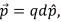where d is the distance between the charges. From simple geometry, it can be seen that,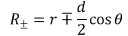Thus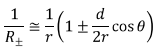so that the potential at a point P is given by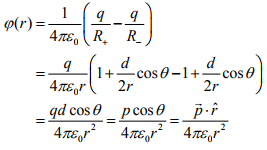The electric field can be calculated from a knowledge of the potential. The gradient operator in spherical polar is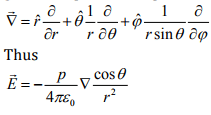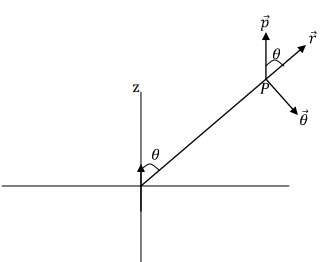The geometry is shown in the figure. It is seen that the unit vector along the dipole moment (taken along the z axis) can be written as,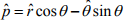so that,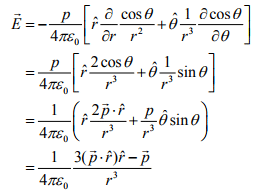This is the dipole field in a coordinate independent form. The lines of force due to a dipole is shown below :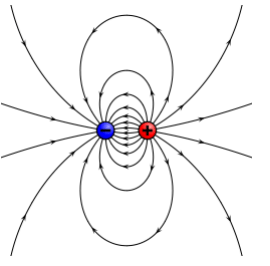Tutorial Assignment

1. We have seen that the potential due to a point charge located at the origin at the position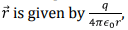where the zero of the potential is taken at infinity. How would this expression change if the zero of the potential was taken on a sphere of radius R about the origin?

2. The electric field in a cubical region of space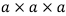is given by the following expression :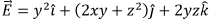verify that this expression can represent an electrostatic field and determine the charge density and the total charge that gives rise to this electric field.

3. A spherical charge distribution is given by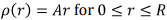and zero outside this region. Obtain a potential corresponding to this distribution.

Solutions to Tutorial Assignment

1. We have to take the line integral of the electric field from r=R to the point in question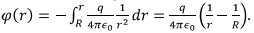2. It can be verified that the curl of the electric field is zero so that the given expression represents a valid electrostatic field. The divergence of the field is given by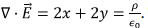Thus the total charge is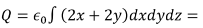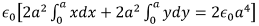3. The electric field is spherically symmetric.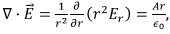which gives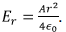(There is an additional tem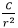possible but we take C=0 so that field does not diverge at r=0. Alternatively, you can use Gauss’s law to arrive at the correct expression for the electric field). The potential corresponding to this field is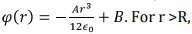the charge density being zero, the potential is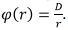Continuity of the potential at r=R gives , for r < R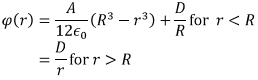Self Assessment Quiz

1. Verify if the following field can represent an electrostatic field in a given region.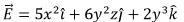If yes, find a potential function and determine the charge density at the point (2,3,0).

2. The electric field in certain region of space is given in spherical polar coordinates as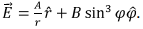Determine the charge density.

3. Find the electric field of a dipole on a point (a) along its axis and (b) along its perpendicular bisector in Cartesian coordinates.

4. Show that the magnitude of the electric field of a dipole at an angle θ to the axis of the dipole is given by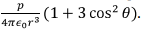Solutions to Self Assessment Quiz

1. The curl of the field is zero and hence the field can be electrostatic. The potential function is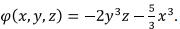The divergence of the field is given by 10x + 12yz which is equal to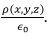Thus at (2,3,0) the charge density is 20∈0.

2.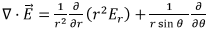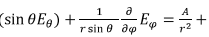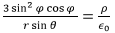3. We have shown that the field of an electric dipole at an angle θ to the axis of the dipole is given by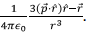Taking the axis along the z axis θ = 0, along the axis so that the field is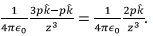Perpendicular to the axis (take it as x direction,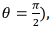the field is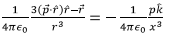4. This can be solved in a straightforward manner.

The document Examples: Electric Field & Potential - Notes | Study Electromagnetic Fields Theory (EMFT) - Electrical Engineering (EE) is a part of the Electrical Engineering (EE) Course Electromagnetic Fields Theory (EMFT).
All you need of Electrical Engineering (EE) at this link: Electrical Engineering (EE)

## Electromagnetic Fields Theory (EMFT)

11 videos|46 docs|62 tests

## Electromagnetic Fields Theory (EMFT)

11 videos|46 docs|62 tests

Track your progress, build streaks, highlight & save important lessons and more!

,

,

,

,

,

,

,

,

,

,

,

,

,

,

,

,

,

,

,

,

,

;